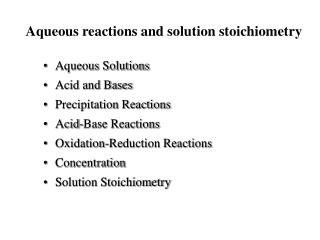DownloadDownload PresentationAqueous reactions and solution stoichiometry

# Aqueous reactions and solution stoichiometry

Télécharger la présentation## Aqueous reactions and solution stoichiometry

- - - - - - - - - - - - - - - - - - - - - - - - - - - E N D - - - - - - - - - - - - - - - - - - - - - - - - - - -
##### Presentation Transcript

1. Aqueous reactions and solution stoichiometry • Aqueous Solutions • Acid and Bases • Precipitation Reactions • Acid-Base Reactions • Oxidation-Reduction Reactions • Concentration • Solution Stoichiometry

2. Solutions • Solution : A homogeneous mixture of two or more components, consists of two component types • Solvent: major component • Solute: component in the lesser extent • Aqueous Solutions : • Ionic compounds • Molecular compounds • Electrolyte : • Strong electrolytes: strong acid/base, soluble ionic compounds • Weak electrolytes: weak acid/base • Nonelectrolytes

3. H2O Acid and Base • Acid - a compound that increases [H+] in water ex. HNO3 H+(aq) + NO3-(aq) • Strong acid: HCl, HBr, HI, HNO3, H2SO4, HClO4, HClO3 • Weak acid • Base - a compound that increases [OH-] in water, proton acceptor • Strong base: hydroxide of IA, IIA, LiOH, NaOH, KOH, RbOH, CsOH, Ca(OH)2, Sr(OH)2, Ba(OH)2 • Weak base

4. Precipitation Reactions • Precipitate • Insoluble product that is formed from a reaction is solution • Solubility: solubility rule, Table 4.1, p. 118 • Exchange reaction (metathesis) AX+BY --> AY + BX • * Exchange reaction will occur when one of the products is insoluble solids, gas, weak or nonelectrolyte • Ionic Equation • Molecular equation • Ionic equation Complete ionic equation, net ionic equation

5. Acid-Base Reactions • Neutralization reaction Acid + Base --> Salt + H2O Ex. HCl (aq) + NaOH (aq) --> NaCl (aq) + H2O (l) • Acid-base reaction with gas formation Ex HCl (aq) + NaHCO3 (aq) --> NaCl (aq) +H2O (l) + CO2 (g)

6. Oxidation-Reduction Reactions • Redox reactions: electrons are transferred between reactants • Oxidation: lose electron • Reduction: gain electron • Oxidation state/oxidation number • Assigning oxidation number • Element • Monatomic ion • Nonmetal in compounds • All other • Displacement reaction A + BX --> AX + B • Activity Series: Table 4.5, P.131

7. Concentration no of moles of solute total volume solution in L Molarity, M = • Concentration: measures how much solute is in a solution • Molarity, M: unit in mol/L • Dilution Mconcx Vconc = Mdil x Vdil

8. Solution Stoichiometry • Procedure: Fig 4.18, P. 139 • Start with balancing equation • Work with moles • Convert to concentration or volume

9. Titration • Titration : A method to determine the amount of a substance in solution • Standard solution (titrant): a solution of known concentration • Equivalence point : when just enough titrant is added to the solution • Indicator : a substance changes color at the end point • End point : when indicator changes color • Acid-base titration: to determine the concentration of unknown solution